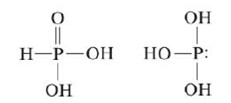Chapter 20, Problem 100CP

Chapter
Section
Textbook Problem

# Many structures of phosphorus-containing compounds are drawn with some P9O bonds. These bonds are not the typical π bonds we’ve considered, which involve the overlap of two p orbitals. Instead, they result from the overlap of a d orbital on the phosphorus atom with a p orbital on oxygen. This type of π bonding is sometimes used as an explanation for why H3PO3 has the first structure below rather than the second:Draw a picture showing how a d orbital and a p orbital overlap to form a π bond.

Interpretation Introduction

Interpretation: Two structures of H3PO3 is given. A picture is to be drawn to show the overlapping of d orbital and p orbital in the first structure to form π bond.

Concept introduction: The bond that is formed due to side to side overlap is known as pie bond.

To determine: A pictorial representation to show the overlapping of d orbital and p orbital in the first structure of H3PO3 to form π bond

Explanation

Explanation

The given structure of H3PO3 is,

Figure 1

The atomic number of central atom phosphorous (P) is 15 and its electronic configuration is,

1s22s22p63s23p3

The valence electron of phosphorous is 5

The atomic number of oxygen (O) is 8 and its electronic configuration is,

1s22s22p4

The valence electron of oxygen is 6 .

The atomic number of hydrogen is 1 and its electronic configuration is,

1s1

The valence electron of hydrogen is 1 .

The formula of number of electron pairs is,

X=V+M±C2

Where,

• X is the number of electron pairs.
• V is the valence electrons of central atom

### Still sussing out bartleby?

Check out a sample textbook solution.

See a sample solution

#### The Solution to Your Study Problems

Bartleby provides explanations to thousands of textbook problems written by our experts, many with advanced degrees!

Get Started